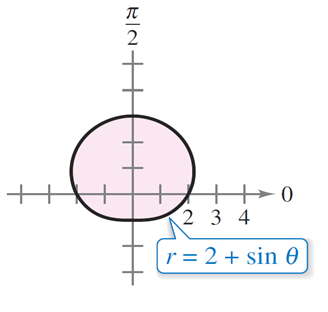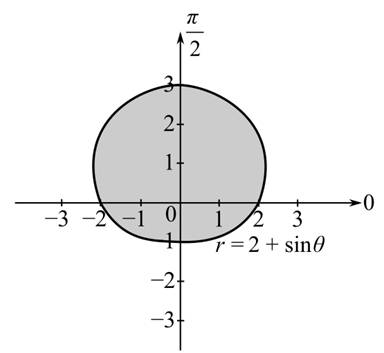Chapter 14.3, Problem 44E

Chapter
Section
Textbook Problem

AreaIn Exercises 41–46, use a double integral to find the area of the shaded region.To determine

To calculate: The area of the shaded region of the figure using the double integration.Explanation

Given:

The figure is

The expression of r is r=2+sinθ.

Formula used:

The integration of:

xndx=xn+1n+1+Csin(ax)dx=cos(ax)a+C.

Calculation:

From the figure, it can be observe that the radius of the curve varies from zero to 2+sinθ that means, 0r2+sinθ

For the variation of the angle θ,

For r=2,

2=2+sinθsinθ=0θ=0,2π

The curve has a period of 2π. The value of θ is, 0θ2π

From obtained data, setup the double integral in polar coordinates for the area of the shaded region:

The expression for the double integral is,

A=02π02+sinθrdrdθ=02π[r22]02+sinθdθ=

Still sussing out bartleby?

Check out a sample textbook solution.

See a sample solution

The Solution to Your Study Problems

Bartleby provides explanations to thousands of textbook problems written by our experts, many with advanced degrees!

Get Started

Distance Find the distance between the given numbers. 73.

Precalculus: Mathematics for Calculus (Standalone Book)

In Exercises 4143, find the distance between the two points. 42. (9, 6) and (6, 2)

Applied Calculus for the Managerial, Life, and Social Sciences: A Brief Approach

In Problems 27 – 30, solve for y in terms of x. 30.

Mathematical Applications for the Management, Life, and Social Sciences

In how many ways can four students be seated in a row of four seats?

Finite Mathematics for the Managerial, Life, and Social Sciences

Find for defined implicity by .

Study Guide for Stewart's Multivariable Calculus, 8th

The average value of f(x) = 3x2 + 1 on the interval [2, 4] is: a) 29 b) 66 c) 58 d) 36

Study Guide for Stewart's Single Variable Calculus: Early Transcendentals, 8th

Describe how replication protects against fraud being committed in research.

Research Methods for the Behavioral Sciences (MindTap Course List)